# Solving Equations Literal Equations with Fractions Graphs of

• Slides: 57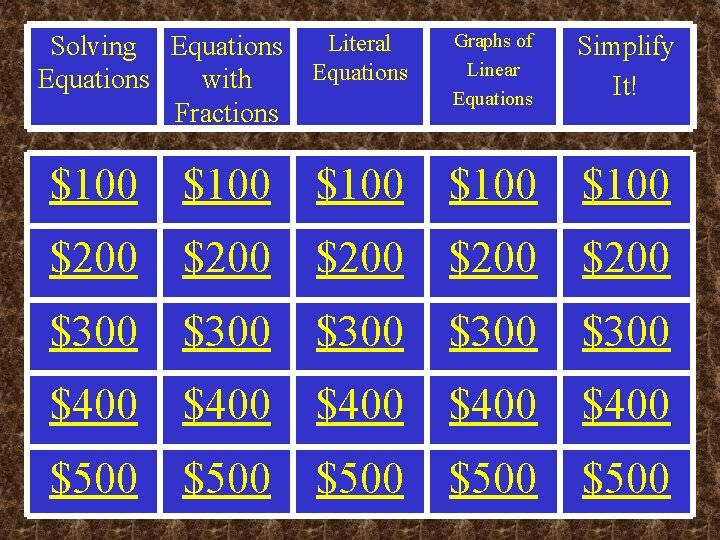Solving Equations Literal Equations with Fractions Graphs of Linear Equations Simplify It! \$100 \$100 \$200 \$200 \$300 \$300 \$400 \$400 \$500 \$500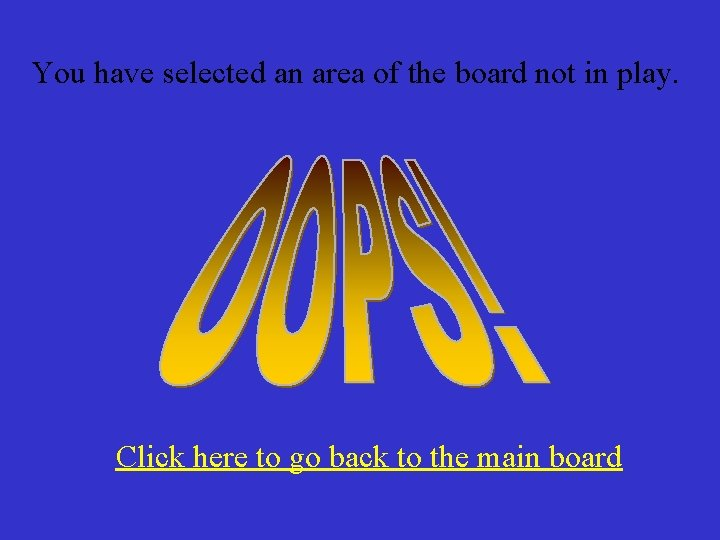You have selected an area of the board not in play. Click here to go back to the main boardSolving Equations - \$100 2 x + 4 = 6 ANSWERSolving Equations - \$200 x/3 – 6 = -3 ANSWERSolving Equations - \$300 4 y – 6 = 2 y + 8 ANSWER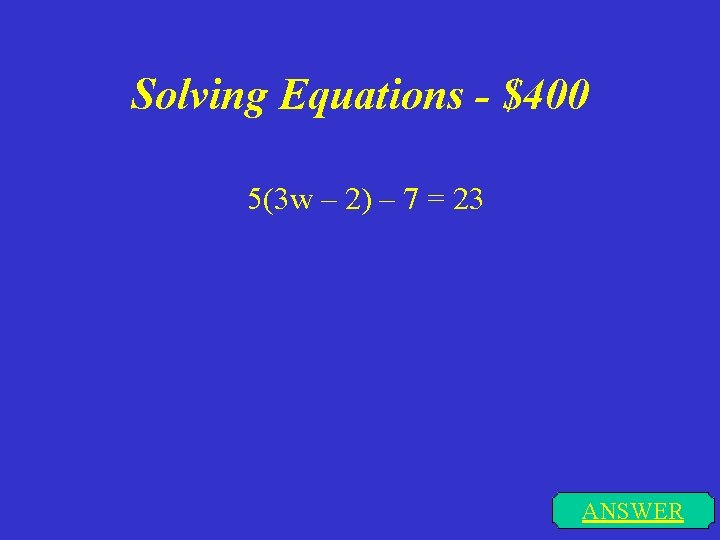Solving Equations - \$400 5(3 w – 2) – 7 = 23 ANSWER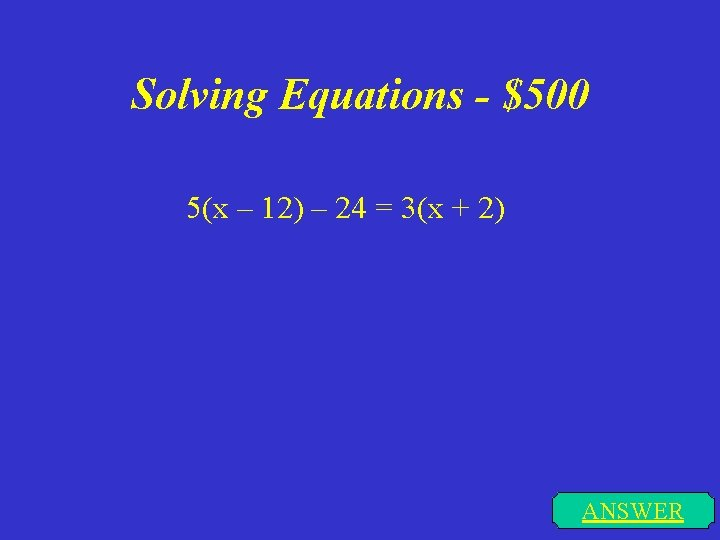Solving Equations - \$500 5(x – 12) – 24 = 3(x + 2) ANSWER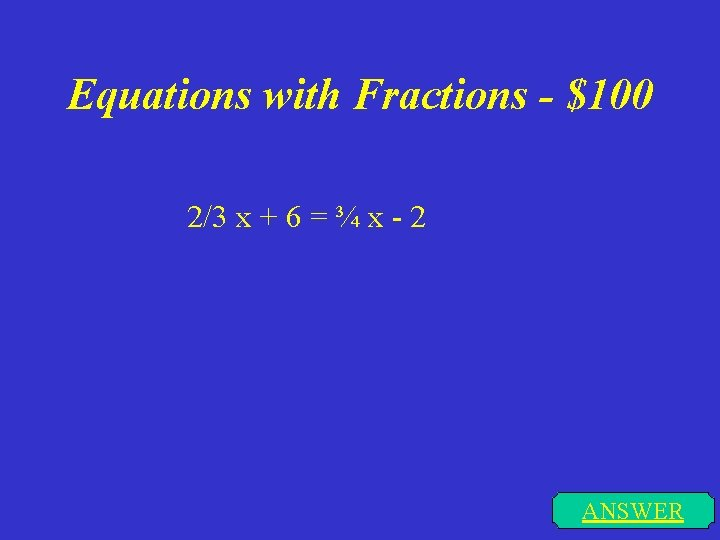Equations with Fractions - \$100 2/3 x + 6 = ¾ x - 2 ANSWEREquations with Fractions - \$200 4/5 x + 1/5 = 2 x -3 ANSWEREquations with Fractions - \$300 1/7 x – 3 = 3/14 x - 1 ANSWER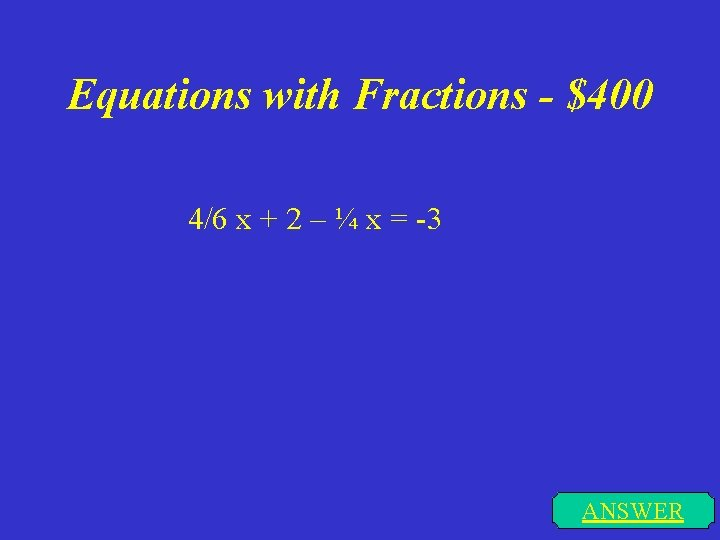Equations with Fractions - \$400 4/6 x + 2 – ¼ x = -3 ANSWEREquations with Fractions - \$500 2/5 x = 1/3 x + 1/5 ANSWERLiteral Equations - \$100 ANSWER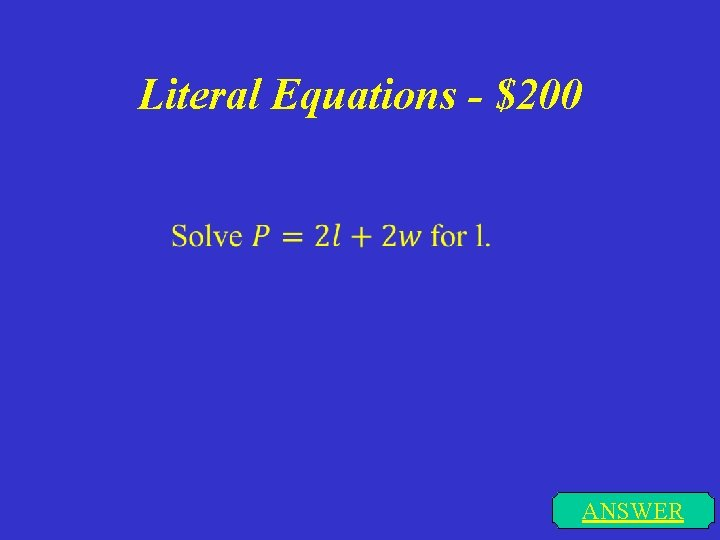Literal Equations - \$200 ANSWER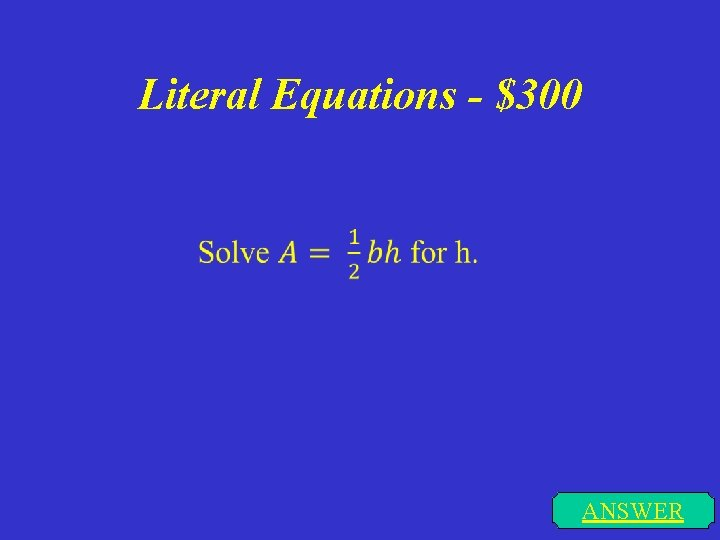Literal Equations - \$300 ANSWER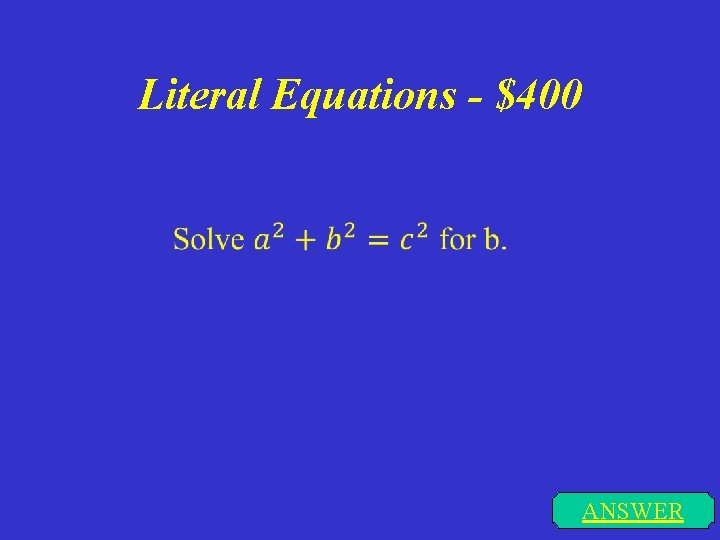Literal Equations - \$400 ANSWERLiteral Equations - \$500 ANSWERGraphs of Linear Equations \$100 Graph y = 2 x - 4 ANSWER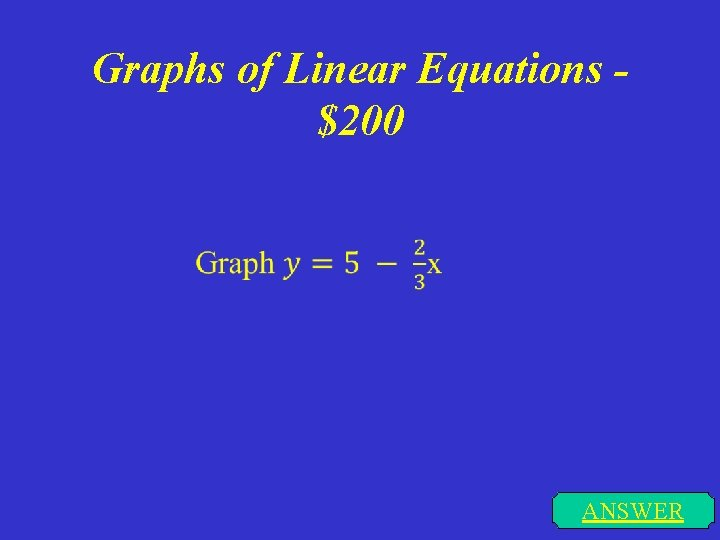Graphs of Linear Equations \$200 ANSWERGraphs of Linear Equations \$300 Graph 3 x – 5 y = 15 ANSWER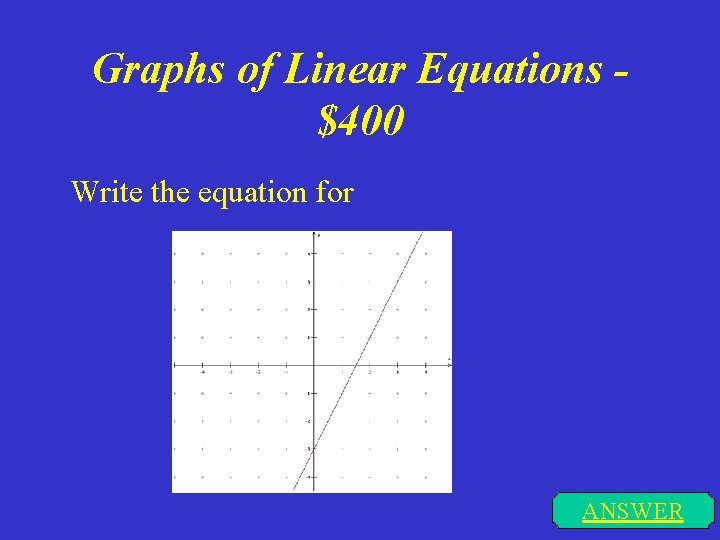Graphs of Linear Equations \$400 Write the equation for ANSWER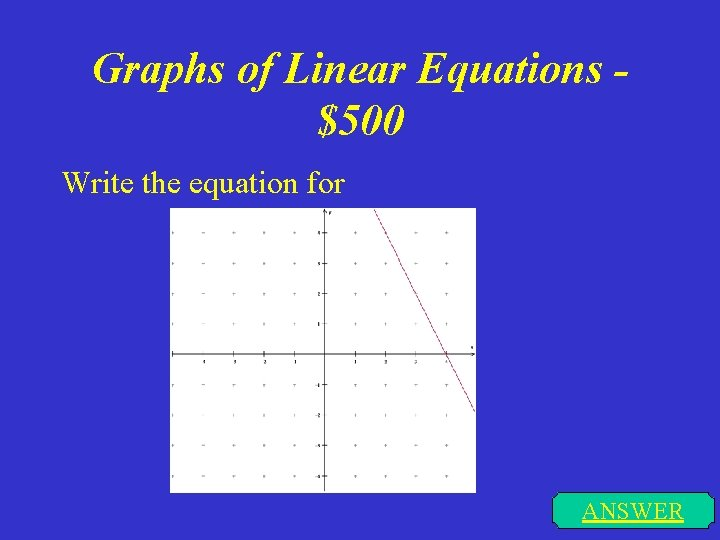Graphs of Linear Equations \$500 Write the equation for ANSWERSimplify It! - \$100 ANSWER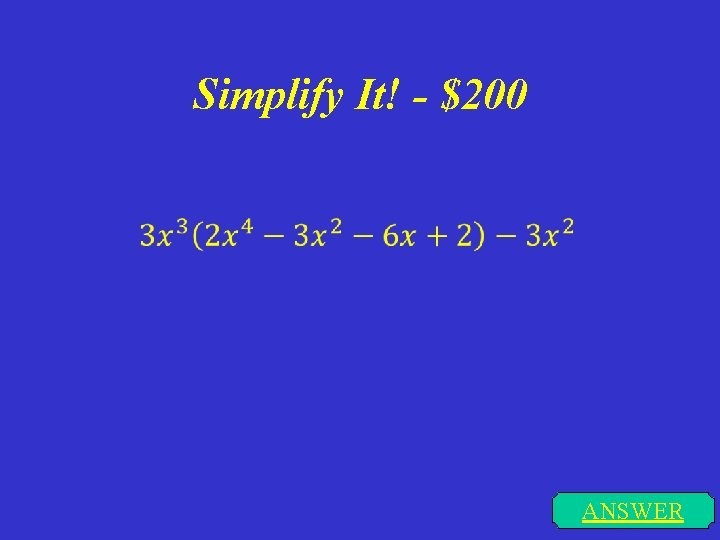Simplify It! - \$200 ANSWERSimplify It! - \$300 ANSWER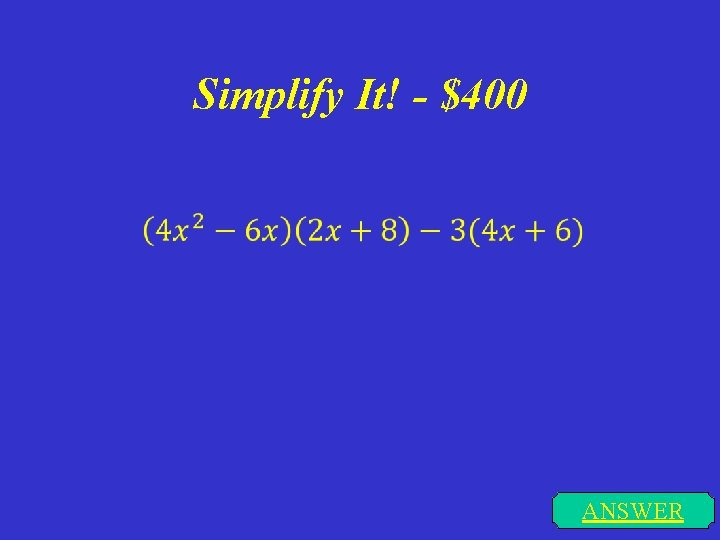Simplify It! - \$400 ANSWER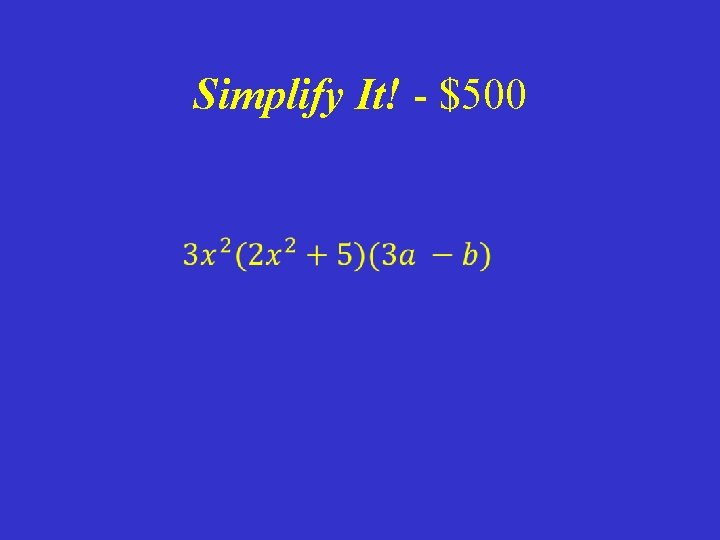Simplify It! - \$500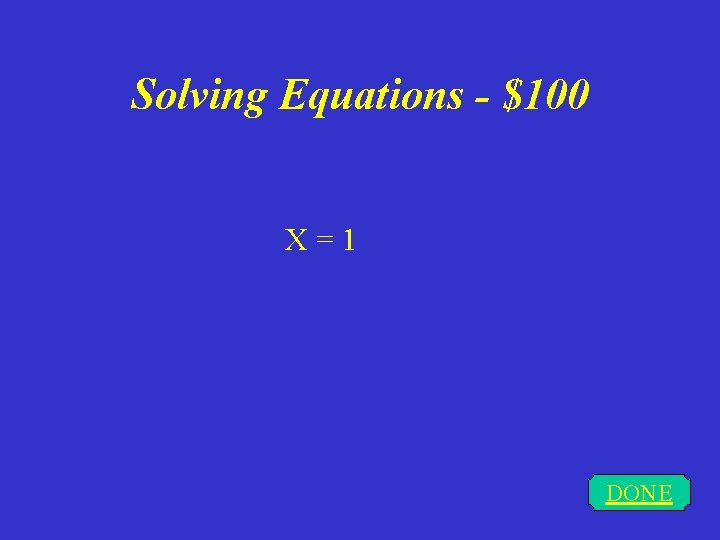Solving Equations - \$100 X = 1 DONESolving Equations - \$200 X = 9 DONE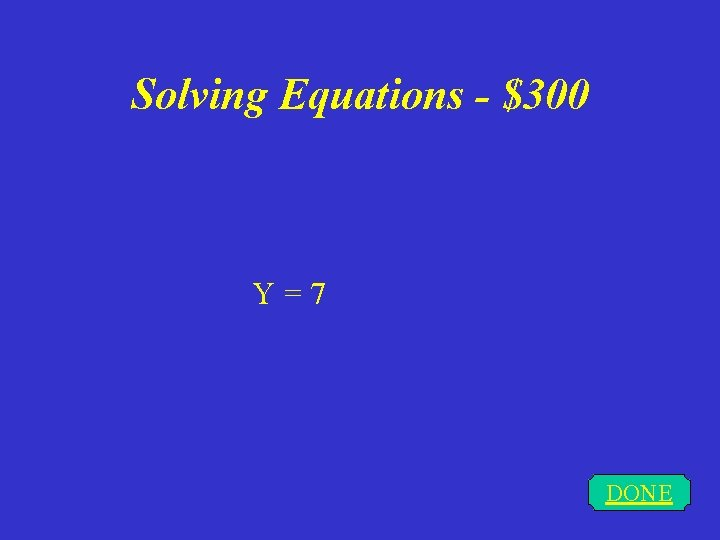Solving Equations - \$300 Y = 7 DONE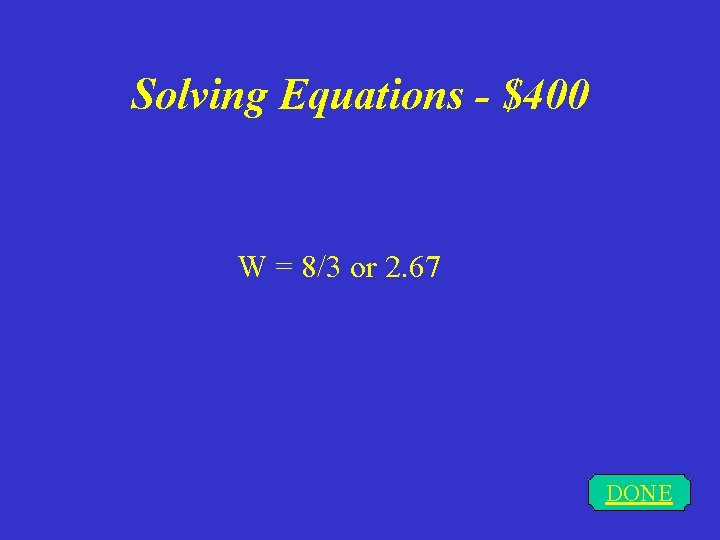Solving Equations - \$400 W = 8/3 or 2. 67 DONESolving Equations - \$500 X = 45 DONEEquations with Fractions - \$100 x = 96 DONEEquations with Fractions - \$200 X=8/3 or 2. 67 DONEEquations with Fractions - \$300 x = -28 DONE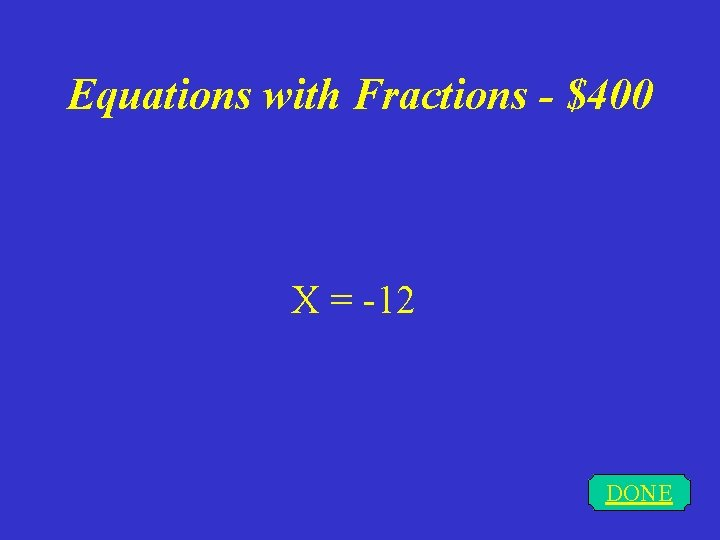Equations with Fractions - \$400 X = -12 DONEEquations with Fractions - \$500 x = 3 DONE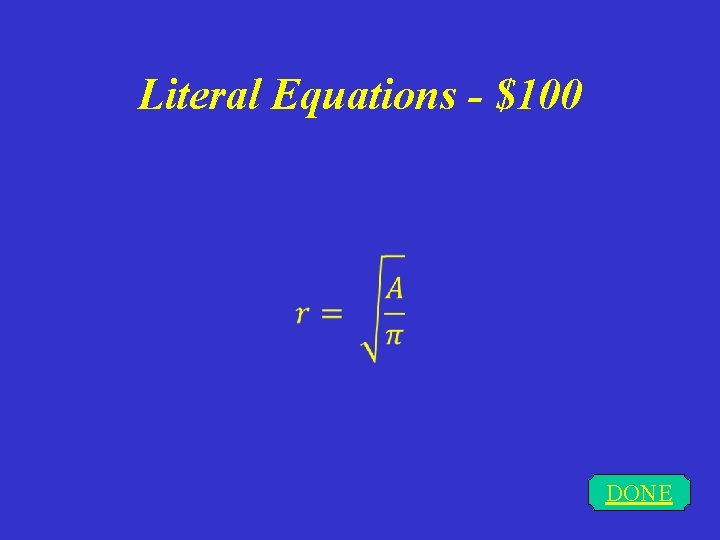Literal Equations - \$100 DONELiteral Equations - \$200 DONE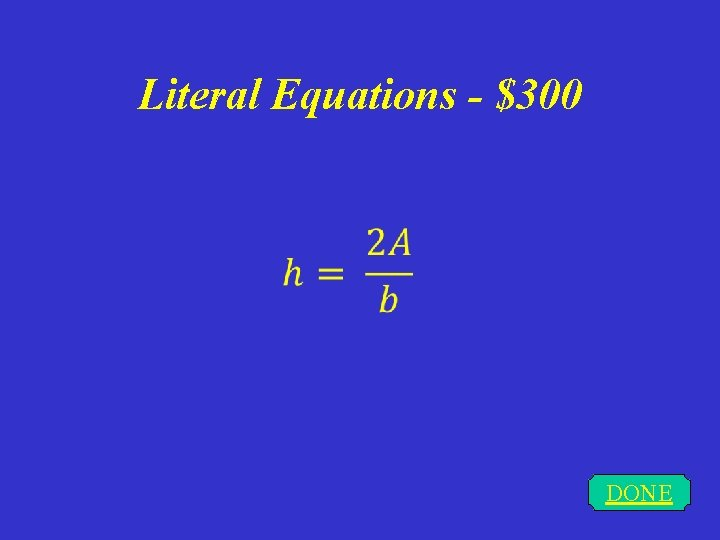Literal Equations - \$300 DONE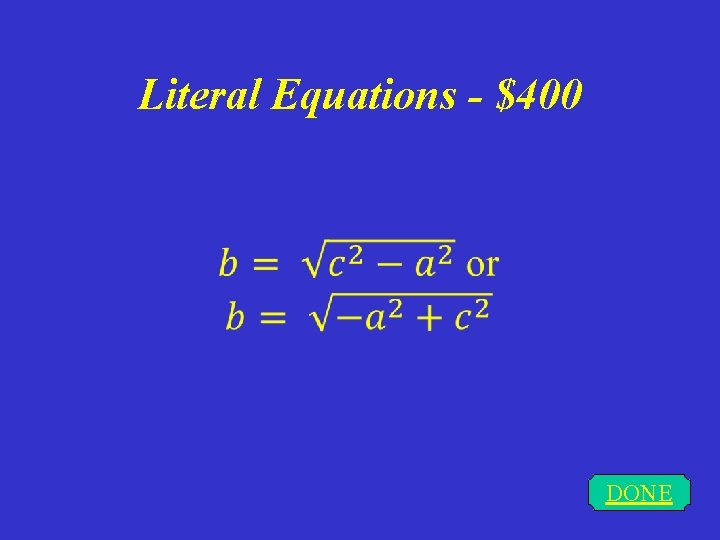Literal Equations - \$400 DONELiteral Equations - \$500 DONE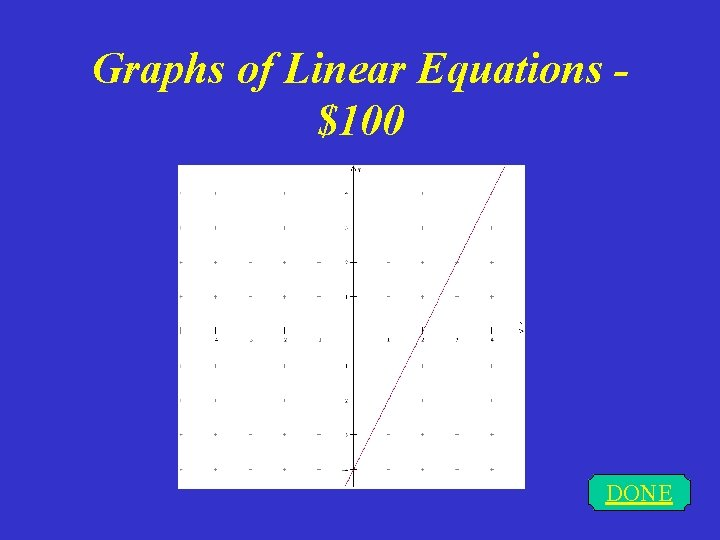Graphs of Linear Equations \$100 DONE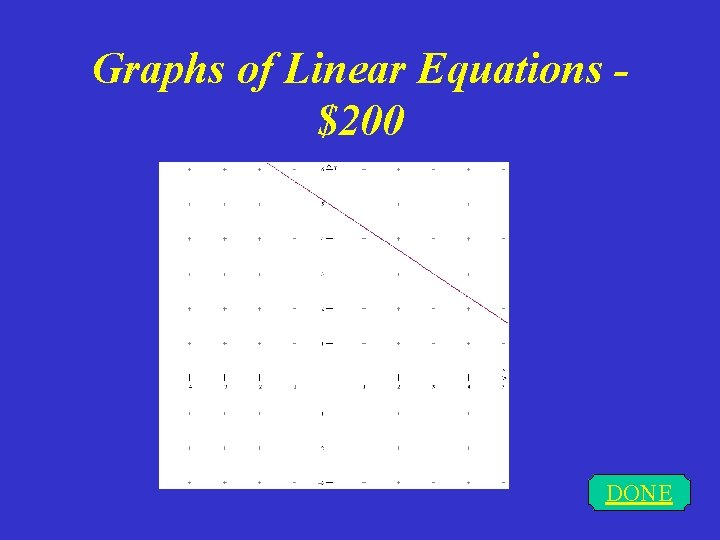Graphs of Linear Equations \$200 DONE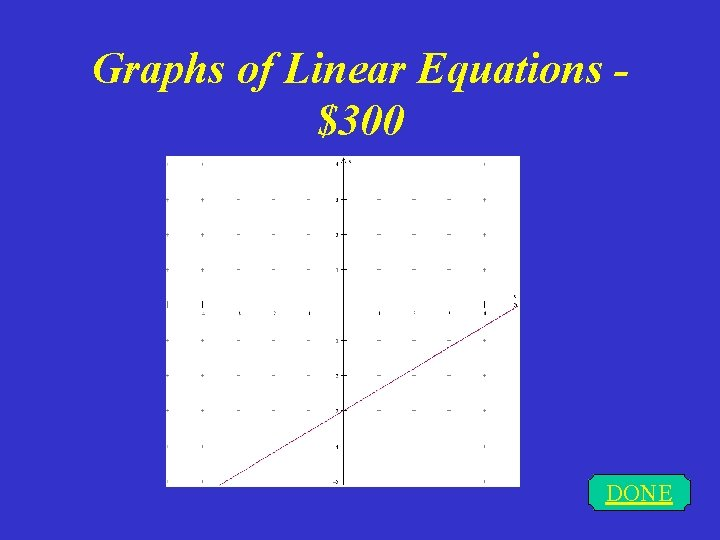Graphs of Linear Equations \$300 DONEGraphs of Linear Equations \$400 DONE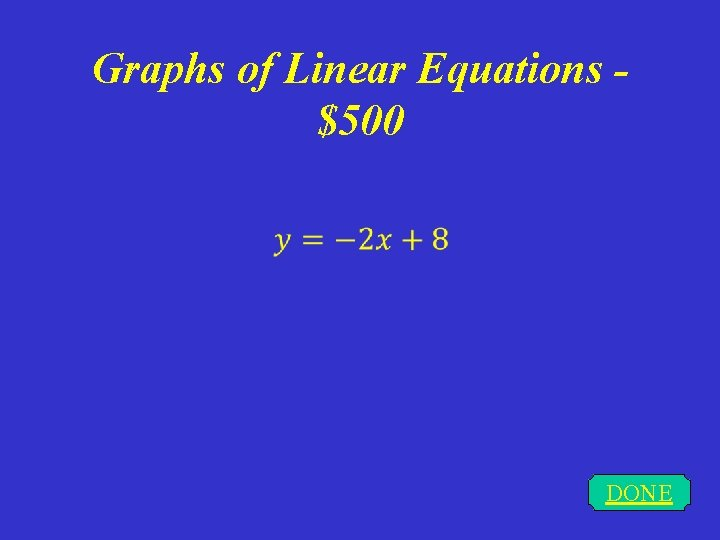Graphs of Linear Equations \$500 DONESimplify It! - \$100 DONE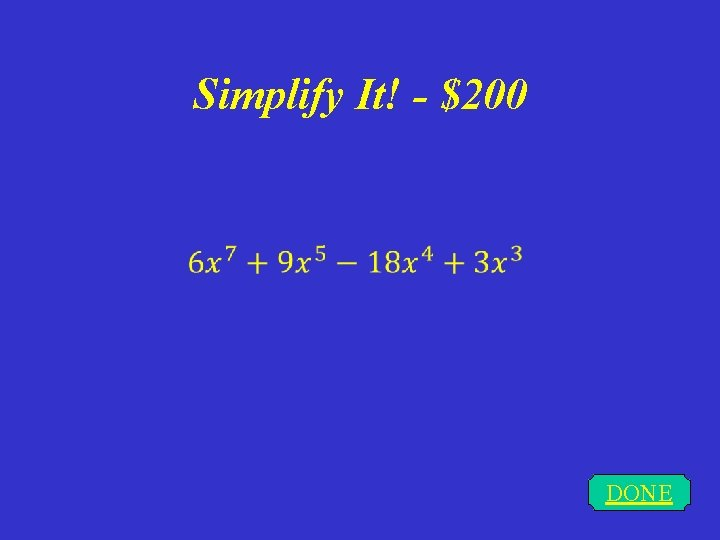Simplify It! - \$200 DONE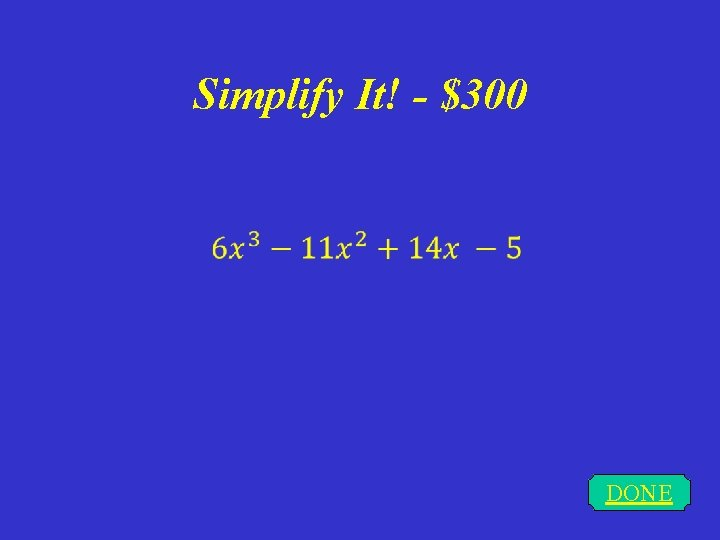Simplify It! - \$300 DONE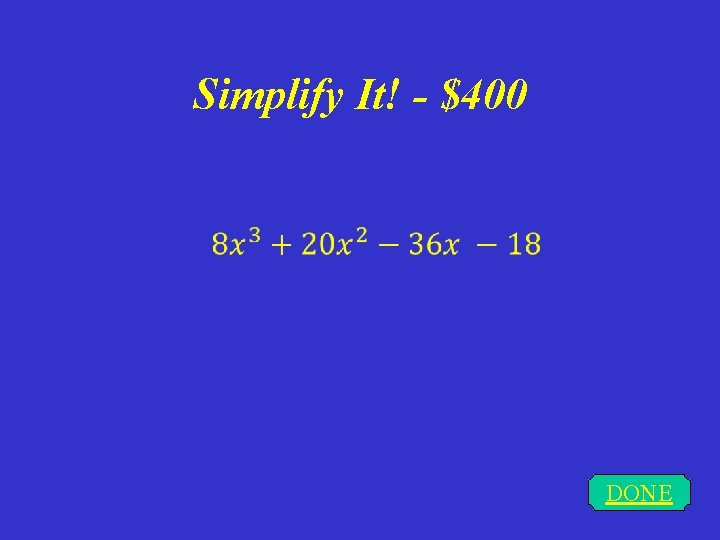Simplify It! - \$400 DONE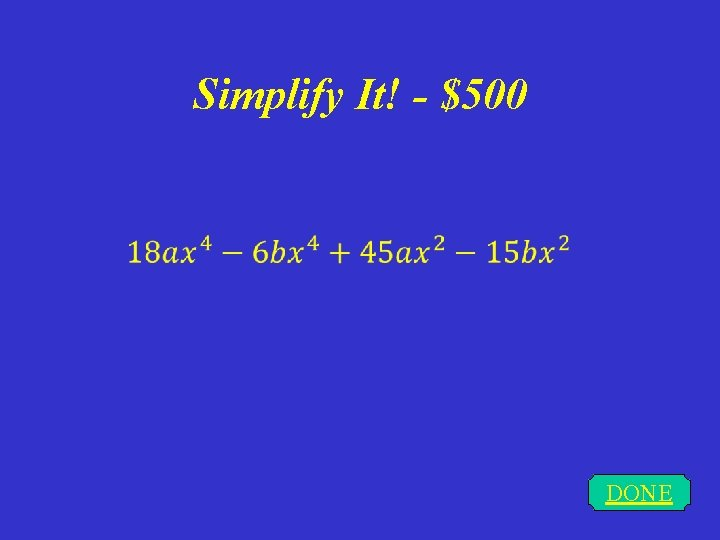Simplify It! - \$500 DONECONTINUECONTINUEDirections for Changing the Game • To change the questions and answers, just type over the problems…Use the “replace” feature to change the categories easily • The daily doubles were originally set to category #4 for \$500 and category #2 for \$300 • To change the daily doubles you must – 1. Change the hyperlink for the links on the main board to go to the appropriate question, therefore bypassing the daily double slide – 2. Change the hyperlink on the continue button on each daily double slide to go to the new question.